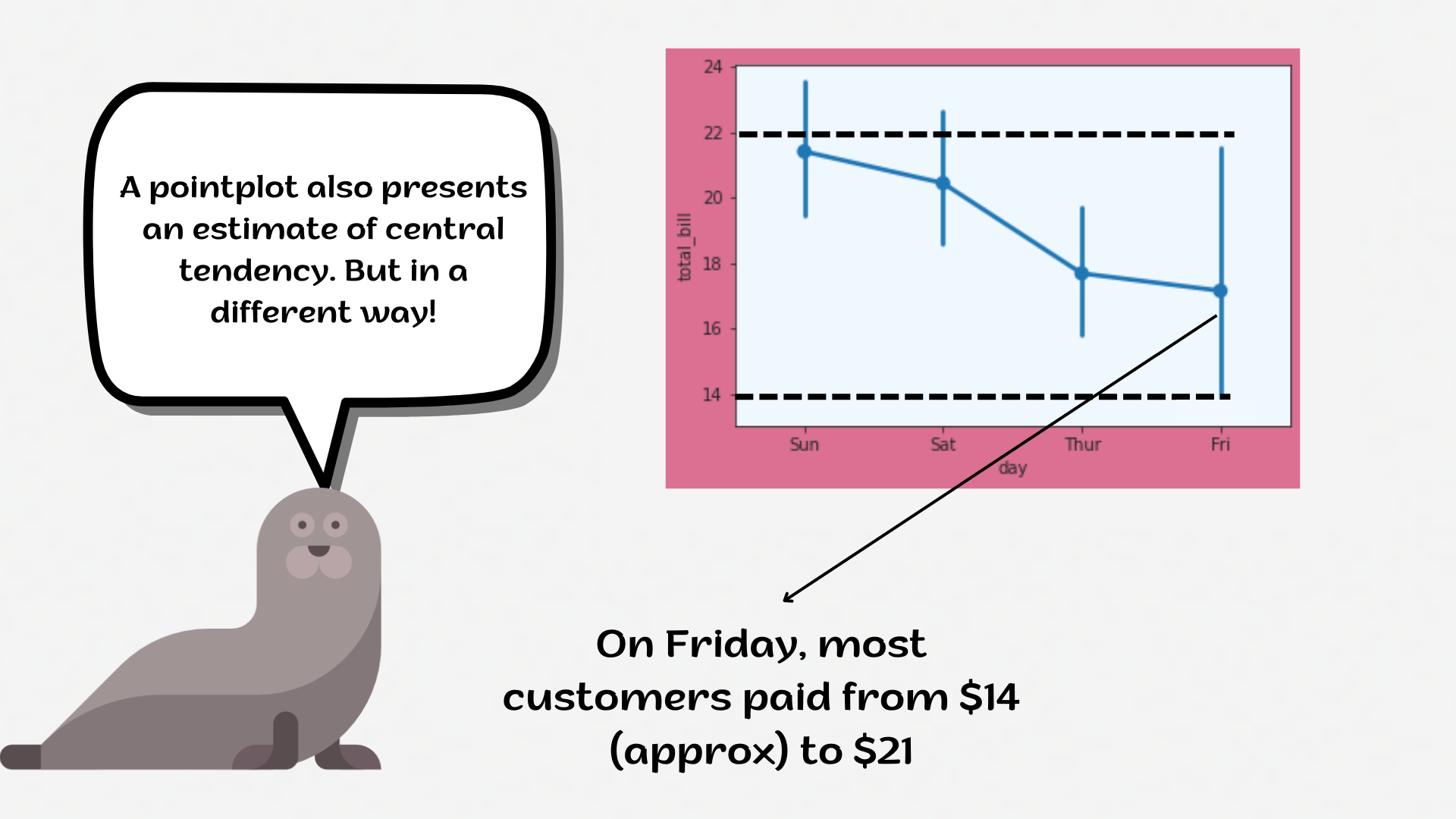Course Content

# Deep Dive into the seaborn Visualization

1. Light Start

2. Distributions of Data

3. Categorical Plot Types

4. Matrix Plots

5. Multi-Plot Grids

6. Regression Models

Deep Dive into the seaborn Visualization

##PointplotA `pointplot` represents an estimate of the central tendency for a numeric variable by the position of scatter plot points and provides some indication of the uncertainty around that estimate using error bars.1. Set the `'ticks'` style with the `'azure'` `axes.facecolor`.
2. Create the `pointplot` using the `seaborn` library and the `g` variable:
• Set the `x` parameter equals the `'day'`;
• Set the `y` parameter equals the `'tip'`;
• Set the `hue` parameter equals the `'sex'`;
• Set `'v'` & `'o'` `markers`;
• Set the `'rocket'` `palette`;
• Set the `dodge` parameter equals the `True`;
• Set the `capsize` parameter equals `0.2`;
• Set the next `linestyles`: `'-'` & `'- -'`;
• Set the data;
• Display the plot.

Everything was clear?

Section 3. Chapter 6